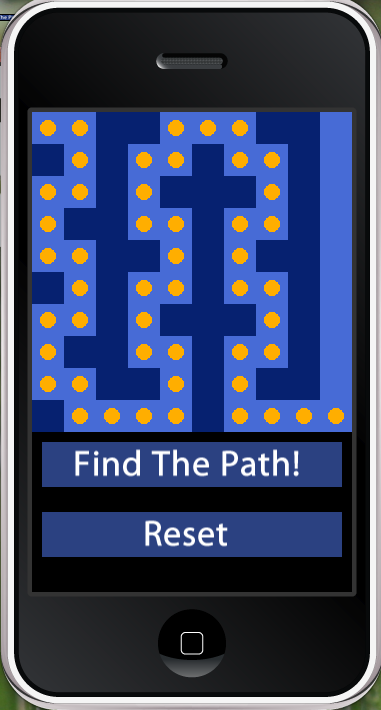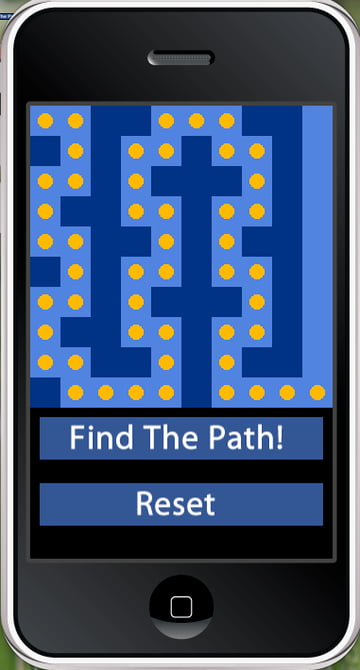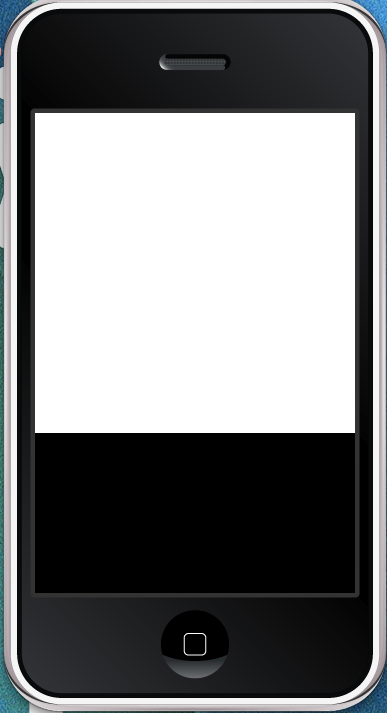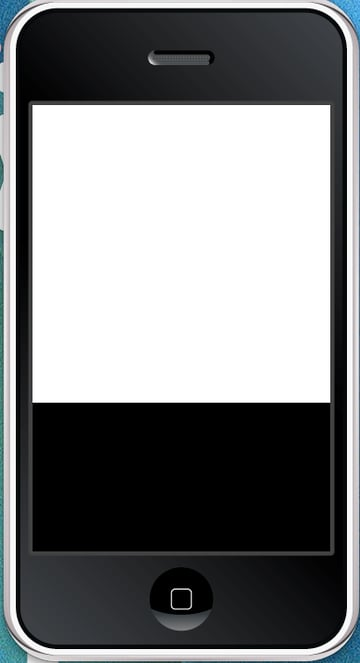# Corona SDK: Game Development Path FindingThis project steps through the basics of path finding in the Corona SDK via the A* path finding algorithm, a staple in the game industry since about 1970. This app lets you add obstacles to a 10x10 grid and view the path determined by A*.## Overview of the A* Algorithm

A* uses two functions to determine the path of "least cost" to the target. The first function, often called g(x), is the cost of moving between two adjacent squares. This is useful when your game has varying terrains such as swamps and paved roads that may have different movement effects. The second function, h(x), is an estimate of the distance from the current square to the target square along the ideal path. There are many different ways to calculate this distance, but for this program, we will utilize a relatively simple approach by adding remaining distance in the y direction to the remaining distance in the x direction.

We will use the following two lua functions to find the path. The function is called as follows, CalcPath(CalcMoves(board, startX, startY, targetX, targetY)). The CalcPath function returns a table of the coordinates to the target, or nil if no path could be found. This behavior will prove helpful in determining the validity of obstacle placement.

If you have no interest in the behind the scenes aspects of the algorithm, feel free to just copy and paste the functions from the full source at the top of the page. Otherwise, fasten your seatbelts: this may be a lot to take in at first.

## Step 1: The First Path Finding Function

Let's take a look at CalcMoves first.

### Declaring Variables

 1 2 local openlist = {} --Possible Moves  3 local closedlist = {} --Checked Squares  4 local listk = 1 -- openlist counter  5 local closedk = 0 -- closedlist counter  6 local tempH = math.abs(startX-targetX) + math.abs(startY-targetY) --h(x)  7 local tempG = 0 

Here we declare most of the variables used in the method.

• openlist is a table that holds all of the possible moves from the current square (up, down, left, right in our example)
• The second table is the closedlist, a list of squares that have already been checked (squares that are part of the path thus far)
• Listk and closedk are counters for the open list and closed list respectively
• tempH and tempG are temporary variables that hold the values of h(x) and g(x) for the current square. The value of tempH is calculated by adding the difference of the starting and target x/y values.
 1 2 openlist = {x = startX, y = startY, g = 0, h = tempH, f = 0 + tempH, par = 1}  3 local xsize = table.getn(board)  4 local ysize = table.getn(board)  5 local curSquare = {}  6 local curSquareIndex = 1 -- Index of current base 

Diving into the next chunk, we begin by setting the first sqare in the open list to the starting square. Then we create variables for the width and height of the board, declare a table for the current square being checked in the open list, and create a variable for the index in the open list of the current square. In case you were wondering, "Par" holds the index of the square's parent. This allows for easy reconstruction of the path.

### Finding the Square of Least Cost

 1 2 while listk > 0 do  3  local lowestF = openlist[listk].f  4  curSquareIndex = listk  5  for k = listk, 1, -1 do  6  if openlist[k].f < lowestF then  7  lowestF = openlist[k].f  8  curSquareIndex = k  9  end  10  end  11 ... 

This method is sort of turned on its head. It loops through, first iterating through the open list, and second expanding the open list until it reaches the final square. The first nested loop iterates through the open list to find the square with the lowest F value. The f value is similar to the h value, except that the f value is the cost of the shortest path from start to end passing through the current square. The index of this square is stored in a variable. Note: If the open list ever becomes empty (i.e. There were no more spaces eligible for movement) a path could not be determined and the function returns nil. This possibility is checked in the while loop.

 1 2 closedk = closedk + 1  3 table.insert(closedlist,closedk,openlist[curSquareIndex])  4 5 curSquare = closedlist[closedk] 

Here we increment the closed list counter, insert the square with the lowest f score into the closed list, and set that square as the current square. The next lines will determine whether the squares adjacent to the new current square are eligible for movement.

### Checking Adjacent Squares for Availability

 1 2 local rightOK = true  3 local leftOK = true -- Booleans defining if they're OK to add  4 local downOK = true -- (must be reset for each while loop)  5 local upOK = true  6 -- Look through closedlist. Makes sure that the path doesn't double back  7 if closedk > 0 then  8  for k = 1, closedk do  9  if closedlist[k].x == curSquare.x + 1 and closedlist[k].y == curSquare.y then  10  rightOK = false  11  end  12  if closedlist[k].x == curSquare.x-1 and closedlist[k].y == curSquare.y then  13  leftOK = false  14  end  15  if closedlist[k].x == curSquare.x and closedlist[k].y == curSquare.y + 1 then  16  downOK = false  17  end  18  if closedlist[k].x == curSquare.x and closedlist[k].y == curSquare.y - 1 then  19  upOK = false  20  end  21  end  22 end 

First, we check if they are already in the closed list:

 1   2 if curSquare.x + 1 > xsize then  3  rightOK = false  4 end  5 6 if curSquare.x - 1 < 1 then  7  leftOK = false  8 end  9 10 if curSquare.y + 1 > ysize then  11  downOK = false  12 end  13 14 if curSquare.y - 1 < 1 then  15  upOK = false  16 end 

Second, it ensures that the adjacent squares are within the bounds of the board:

 1 2 if curSquare.x + 1 <= xsize and board[curSquare.x+1][curSquare.y].isObstacle ~= 0 then  3  rightOK = false  4 end  5 6 if curSquare.x - 1 >= 1 and board[curSquare.x-1][curSquare.y].isObstacle ~= 0 then  7  leftOK = false  8 end  9 10 if curSquare.y + 1 <= ysize and board[curSquare.x][curSquare.y+1].isObstacle ~= 0 then  11  downOK = false  12 end  13 14 if curSquare.y - 1 >= 1 and board[curSquare.x][curSquare.y-1].isObstacle ~= 0 then  15  upOK = false  16 end 

Third, we check if the adjacent squares contain obstacles:

 1 2 -- check if the move from the current base is shorter then from the former parrent  3 tempG =curSquare.g + 1  4 for k=1,listk do  5  if rightOK and openlist[k].x==curSquare.x+1 and openlist[k].y==curSquare.y and openlist[k].g>tempG then  6  tempH=math.abs((curSquare.x+1)-targetX)+math.abs(curSquare.y-targetY)  7  table.insert(openlist,k,{x=curSquare.x+1, y=curSquare.y, g=tempG, h=tempH, f=tempG+tempH, par=closedk})  8  rightOK=false  9  end  10   11  if leftOK and openlist[k].x==curSquare.x-1 and openlist[k].y==curSquare.y and openlist[k].g>tempG then  12  tempH=math.abs((curSquare.x-1)-targetX)+math.abs(curSquare.y-targetY)  13  table.insert(openlist,k,{x=curSquare.x-1, y=curSquare.y, g=tempG, h=tempH, f=tempG+tempH, par=closedk})  14  leftOK=false  15  end  16 17  if downOK and openlist[k].x==curSquare.x and openlist[k].y==curSquare.y+1 and openlist[k].g>tempG then  18  tempH=math.abs((curSquare.x)-targetX)+math.abs(curSquare.y+1-targetY)  19  table.insert(openlist,k,{x=curSquare.x, y=curSquare.y+1, g=tempG, h=tempH, f=tempG+tempH, par=closedk})  20  downOK=false  21  end  22 23  if upOK and openlist[k].x==curSquare.x and openlist[k].y==curSquare.y-1 and openlist[k].g>tempG then  24  tempH=math.abs((curSquare.x)-targetX)+math.abs(curSquare.y-1-targetY)  25  table.insert(openlist,k,{x=curSquare.x, y=curSquare.y-1, g=tempG, h=tempH, f=tempG+tempH, par=closedk})  26  upOK=false  27  end  28 end 

Finally, the algorithm checks to make sure that it is "cheaper" to move from the current square to the next square than it would be to move from the parent square to the next square. This ensures that the path chosen is indeed the cheapest path possible, and there aren't any obvious shortcuts that were missed.

### Expanding the Open List

 1 2 -- Add point to the right of current point  3 if rightOK then  4  listk=listk+1  5  tempH=math.abs((curSquare.x+1)-targetX)+math.abs(curSquare.y-targetY)  6  table.insert(openlist,listk,{x=curSquare.x+1, y=curSquare.y, g=tempG, h=tempH, f=tempG+tempH, par=closedk})  7 end  8 9 -- Add point to the left of current point  10 if leftOK then  11  listk=listk+1  12  tempH=math.abs((curSquare.x-1)-targetX)+math.abs(curSquare.y-targetY)  13  table.insert(openlist,listk,{x=curSquare.x-1, y=curSquare.y, g=tempG, h=tempH, f=tempG+tempH, par=closedk})  14 end  15 16 -- Add point on the top of current point  17 if downOK then  18  listk=listk+1  19  tempH=math.abs(curSquare.x-targetX)+math.abs((curSquare.y+1)-targetY)  20  table.insert(openlist,listk,{x=curSquare.x, y=curSquare.y+1, g=tempG, h=tempH, f=tempG+tempH, par=closedk})  21 end  22 23 -- Add point on the bottom of current point  24 if upOK then  25  listk=listk+1  26  tempH=math.abs(curSquare.x-targetX)+math.abs((curSquare.y-1)-targetY)  27  table.insert(openlist,listk,{x=curSquare.x, y=curSquare.y-1, g=tempG, h=tempH, f=tempG+tempH, par=closedk})  28 end 

This second to last chunk is where we finally expand the open list after all of that arduous testing. If a square adjacent to the current square was able to perservere through our rigorous conditions, it earns a spot on the open list, where it may be chosen as the next current square depending on its F value.

 1 2  ...  3  table.remove(openlist,curSquareIndex)  4  listk=listk-1  5 6  if closedlist[closedk].x==targetX and closedlist[closedk].y==targetY then  7  return closedlist  8  end  9  end  10 11  return nil  12 end 

At last we come to the end of the lengthy function. First we remove the current square from the open list. We then check if the current square's x and y positions equal those of the target square. If so, we pass the closed list to the CalcPath method where the data is refined.

## Step 2: The Second Path Finding Function

 1 2 function CalcPath(closedlist)  3  if closedlist==nil then  4  return nil  5  end  6  local path={}  7  local pathIndex={}  8  local last=table.getn(closedlist)  9  table.insert(pathIndex,1,last)  10 11  local i=1  12  while pathIndex[i]>1 do  13  i=i+1  14  table.insert(pathIndex,i,closedlist[pathIndex[i-1]].par)  15  end  16 17  for n=table.getn(pathIndex),1,-1 do  18  table.insert(path,{x=closedlist[pathIndex[n]].x, y=closedlist[pathIndex[n]].y})  19  end  20 21  closedlist=nil  22  return path  23 end 

It's all downhill from here! This function cuts down the closed list to ensure that only the correct path is returned. Without this if the algorithm traversed a path, got stuck, and struck out on a new path, the table that was returned would contain coordinates for the correct path as well as the failed attempt. All it does is start at the end of the closed list, and reconstruct the path to the beginning via the parent property added earlier. After we have obtained our list of correct coordinates, we can use that information to create a finished path in the second loop. That's all there is to the A* algorithm. Next up we look at how we can use it in a program.

## Step 3: Setting up the Grid

Whew! That was a lot of new information. Luckily the rest of this app is very easy to construct with even a basic understanding of the Corona API. The first thing you should do is create your main.lua file and put it in a new directory. That's all Corona requires in the way of setup! Next we are going to create the table that will hold our 10x10 grid, and While we're at it, we can get rid of that unsightly status bar at the top of the screen.

 1 2 display.setStatusBar(display.HiddenStatusBar)  3 4 board = {} --Create an empty table to hold the board  5 6 --Populate the Table  7 for i = 1, 10 do  8  board[i] = {}  9  for j = 1, 10 do  10  board[i][j] = {}  11  board[i][j].square = display.newRect((i-1) * 32, (j-1) * 32, 32, 32)  12  board[i][j].square:setFillColor(255, 255, 255)  13  board[i][j].isObstacle = 0  14  end  15 end 

There's nothing revolutionary here. All we did was hide the status bar and create a three dimensional array to hold the grid. In the for loops, we set the side length of our squares to 32 pixels and positioned them with some crafty multiplication. Note: Be sure to use the .square and .isObstacle keys instead of just saying board.

Adding obstacles is a simple task. For this tutorial, spaces that are eligible for movement will be white, obstacles will be black, and the markers that indicate the path will be red. Look at the following function:

 1 2 addObstacle = function(event)  3  if event.phase == "ended" and event.y < 320 then  4  --Use event.x and event.y to calculate the coordinate in terms of the 10x10 grid  5  x = math.ceil(event.x/32)  6  y = math.ceil(event.y/32)  7  board[x][y].isObstacle = 1  8  if CalcPath(CalcMoves(board, 1, 1, 10, 10)) then  9  board[x][y].square:setFillColor(0, 0, 0)  10  else  11  board[x][y].isObstacle = 0  12  end  13  end  14  return true  15 end  16 Runtime:addEventListener("touch", addObstacle) 

Notice that the function is a runtime event listener. Runtime events are sent to all listeners and don't apply to a specific object. This is the desired behavior for this implementation. The addObstacle function begins by checking if the user has lifted his finger. Forgetting this step is sure to cause much frustration on the part of the user as the button could be pressed by an accidental swipe, and not a deliberate down and up motion of the finger. Users are accustomed to these nuances, so it is important to pay attention to them whenever possible. This same conditional also makes sure that only touch events that occur within the 320px tall grid are sent to this method. This keeps them from interfering with our buttons.

Besides that, this function uses some basic math to figure out what square was touched using event.y and event.x, and it calls the path finding function to ensure that the user leaves a path. Depending on your needs, this may or may not be desirable, but it's nice to know that you have the ability to make these determinations.## Step 5: Using the Path Finding Information

For the sake of brevity, this tutorial will skimp on animations, and just place markers along the path determined by A*.

 1 2 placeMarkers = function(event)  3  path = CalcPath(CalcMoves(board, 1, 1, 10, 10))  4  for i = 1, table.getn(path) do  5  local newX = path[i].x  6  local newY = path[i].y  7  local marker = display.newCircle((newX*32 - 16), (newY*32 - 16), 8)  8  marker:setFillColor(255, 0, 0)  9  end  10 end 

This is all the code that is necessary. We simply put the coordinates into a table named path, and iterate through the entirety of the table, placing a marker with an eight pixel radius at each set of coordinates. Things can become a little hairy when you attempt to use animations in conjunction with this, but it shouldn't be too bad as long as you keep track of the index of your current coordinate, and increment it after a certain time interval.

## Step 6: Finishing up With Buttons, and Reset Functionality

As it stands currently, the animate function will do absolutely nothing because it's never called. Using a runtime event really isn't appropriate in this instance, so we'll use an image with an event listener.

 1 2 local goButton = display.newImage("PlaceMarkers.png", -200, 350)  3 goButton:addEventListener("touch", placeMarkers) 

Voila! With two lines of code we are set to go.

If you run the program now, you will no doubt notice that the markers don't go away when you run the program again. The solution is to put the nested for loops that initially populated the table into a method that we can call whenever we need a clean slate. The beginning of the program should now look like this:

 1 2 setup = function()  3  counter = 1  4  board = {}  5  --Populate the Table  6  for i = 1, 10 do  7  board[i] = {}  8  for j = 1, 10 do  9  board[i][j] = {}  10  board[i][j].square = display.newRect((i-1) * 32, (j-1) * 32, 32, 32)  11  board[i][j].square:setFillColor(255, 255, 255)  12  board[i][j].isObstacle = 0  13  end  14  end  15 end 

Just two more lines and our program will be complete! I took the liberty of adding a better looking color scheme to the program. I encourage you to play around with this project. See if you can stump it. If you're feeling brave, try modifying the h(x) function and see what happens!

 1 2 local resetButton = display.newImage("Reset.png", 300, 350)  3 resetButton:addEventListener("touch", setup) 

## Conclusion

This tutorial covers a lot of ground! The meat of it was the path finding algorithm. A* is a very powerful tool, and this was a fairly simple version of it. You may have noticed that it didn't account for diagonal movement and was based on squares. The truth is, you can modify the algorithm to accommodate any shape tile, and you can modify h(x) to achieve diagonal movement. The great thing is, while your algorithm code may become complex, the code for the UI and the rest of the application will remain simple and quick to write thanks to the power and simplicity of the Corona SDK. It provides you unprecedented ability in two dimensions without the complexity of Objective-C.